The MCMC Procedure
 PREDDIST Statement

PREDDIST <’label’> OUTPRED=SAS-data-set <NSIM=n> <COVARIATES=SAS-data-set> <STATISTICS=options> ;

The PREDDIST statement creates a new SAS data set that contains random samples from the posterior predictive distribution of the response variable. The posterior predictive distribution is the distribution of unobserved observations (prediction) conditional on the observed data. Let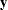be the observed data,be the covariates,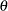be the parameter, and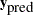be the unobserved data. The posterior predictive distribution is defined to be the following: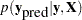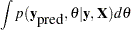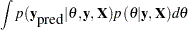Given the assumption that the observed and unobserved data are conditional independent given, the posterior predictive distribution can be further simplified as the following: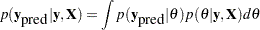The posterior predictive distribution is an integral of the likelihood function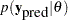with respect to the posterior distribution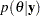. The PREDDIST statement generates samples from a posterior predictive distribution based on draws from the posterior distribution of.

The PREDDIST statement works only on response variables that have standard distributions, and it does not support either the GENERAL or DGENERAL functions. Multiple PREDDIST statements can be specified, and an optional label (specified as a quoted string) helps identify the output.

The following list explains specifications in the PREDDIST statement:

COVARIATES=SAS-data-set

names the SAS data set that contains the sets of explanatory variable values for which the predictions are established. This data set must contain data with the same variable names as are used in the likelihood function. If you omit the COVARIATES= option, the DATA= data set specified in the PROC MCMC statement is used instead.

NSIM=n

specifies the number of simulated predicted values. By default, NSIM= uses the NMC= option value specified in the PROC MCMC statement.

OUTPRED=SAS-data-set

creates an output data set to contain the samples from the posterior predictive distribution. The output variable names are listed as resp_1resp_m, where resp is the name of the response variable and m is the number of observations in the COVARIATES= data set in the PREDDIST statement. If the COVARIATES= data set is not specified, m is the number of observations in the DATA= data set specified in the PROC statement.

STATISTICS<(global-stats-options)> =  NONE | ALL |stats-request
STATS<(global-stats-options)> =  NONE | ALL |stats-request

specifies options for calculating posterior statistics. This option works identically to the STATISTICS= option in the PROC statement. By default, this option takes the specification of the STATISTICS= option in the PROC MCMC statement.

For an example that uses the PREDDIST statement, see Posterior Predictive Distribution.Previous Page | Next Page | Top of Page